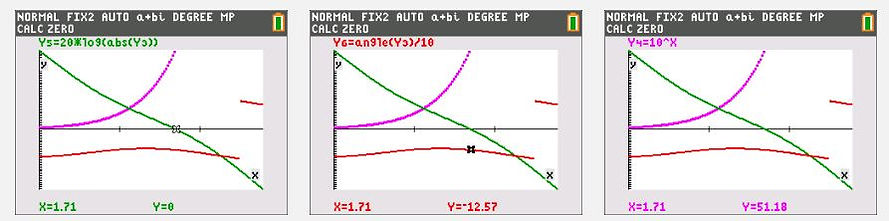naver-site-verification: naver47238a1b6bfbb19a2fd4b619734fa9a6.html
top of page

# Bode diagram program with the Ti-84 Plus (CE) page 2

## PI-control

In Electrical engineering, PI control is a frequently used control principle. The Ti-84 Basic program can help you to gain a better understanding of this method of control. It is known that if only a proportional gain is used, a difference between the actual speed and the desired speed will occur if disturbances are present. Adding a parallel integrator with a time constant of τi to the proportional gain solves this problem for static and low-frequency disturbances.Although the PI controller compensates low-frequency disturbances, it decreases the phase margin (stability) at 0dB.  It can be shown that if taui follows the rule below, the phase margin decreases with about 5 degrees. Kp is the proportional gain for a 60° phase margin (see page 1 of the Bode diagram).taui=1/(0.1*0.52*51.12)=0.38 sec

#### Drawing the Bode diagram of an open loop with PI controller (with Kp=0.52 and taui=0.38 sec} should give a phase margin of approximately 55° (60° - 5°). This results in a phase of -125°.Inserting the PI controller, Hr(s) with Kp=0.52, TAUI=0.38 sec., gives as results a phase margin of 180deg-125.7deg=54.3 deg at 51.18 rad/sec, which is in exact agreement with the Matlab results below.

0dB @ x=1.71=51.18 rad/sec                 Phase margin 180-10*12.57= 54.3deg                x=1.71 equals to 51.18 rad/secBode plot with Matlab

## Closed loop analysis with the  program BODEHOC.8XP

#### At the end, we notice that it is also possible to create a Bode plot of the closed loop system with Kp=0.52 and taui=0.38 sec. The closed loop results :Hc=HrHs/(1+HrHs) are given: Bandwidth : -3 dB  at 86.79 rad sec.  In agreement with Matlab-3dB @ X=1.94                                            X=1.95 equals 86.9 rad/sec .A Bode plot with Matlab showed that at -3 dB, the frequency was 86.7 rad/sec, which

agrees well with the results from the TI-84.